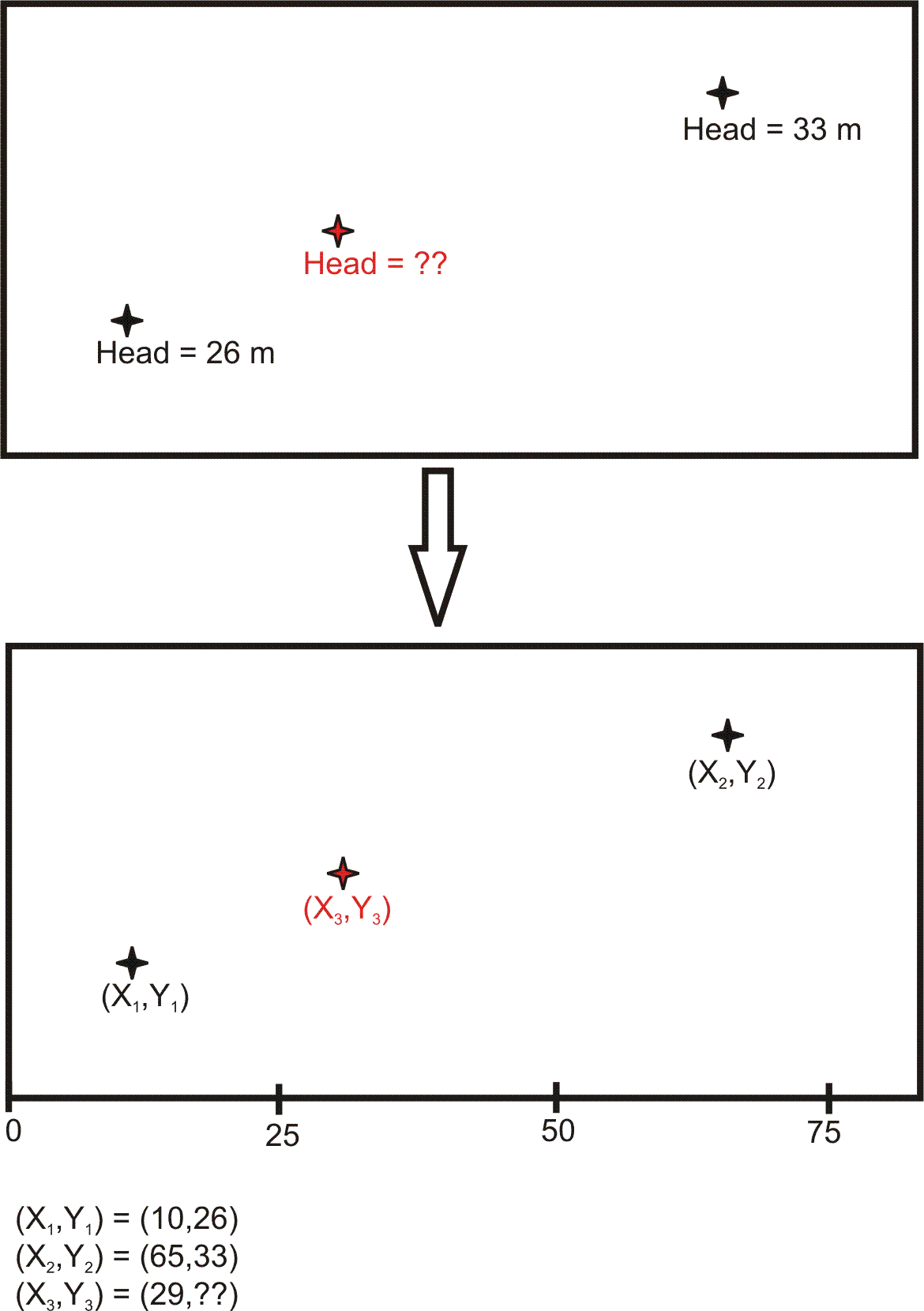## Calculator 2 - Linear Interpolation

###Linear interpolation is one of the simplest ways of interpolation.  Linear interpolation determines, from two points (x1,y1) and (x2,y2), what the value of y is at a different point x3.  Linear interpolation assumes a linear slope between points 1 and 2 and based on that slope will determine what the value of y would be at another given point x.  Obviously a limitation of this method is that it assumes a linear slope, and thus if the parameters do not have a linear relationship the value returned would be incorrect.LINEAR INTERPOLATION CALCULATOR

First Point

X1:
Y1:

Second Point

X2:
Y2:

Third Point

X3:

Y3:EXAMPLE:slope = (33-26)/(65-10) = 0.127

note:  slope is rounded and therefore the following calculation is approximate

Y3 = Y1+ slope x (X3-X1)

= 26 + 0.127*(29-10) = 28.413